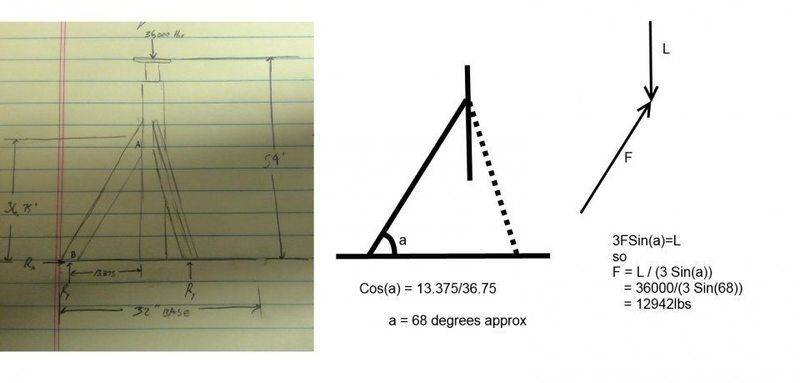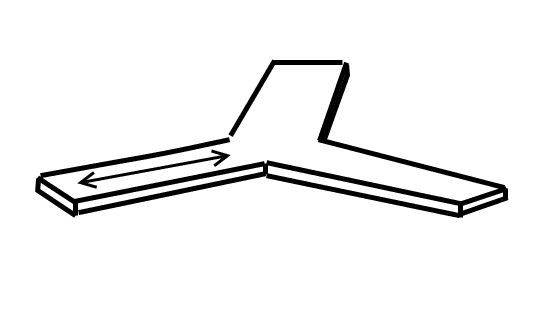# Compression load in jack stand leg

If I have a jack stand that is essentially a tri-pod, with all legs at the base equidistant from the center tube, and a 36,000 pound load on the jack. What is the compression in each leg given these dimensions?
My attempt:

Each reaction in the vertical direction is 1/3 the load, and there would also be a horizontal reaction acting radially inward at each leg. Analyzing one leg, you can find this reaction with summation of moments about point A, and then find the resultant vector (compressive force) by the square of the sum of the squares of the reactions ( sorry it got cut off, but you get the idea). Did I miss anything?

#### Attachments

•IMG_1862.jpg
51.7 KB · Views: 513

SteamKing
Staff Emeritus
Homework Helper
If I have a jack stand that is essentially a tri-pod, with all legs at the base equidistant from the center tube, and a 36,000 pound load on the jack. What is the compression in each leg given these dimensions?
My attempt:

Each reaction in the vertical direction is 1/3 the load, and there would also be a horizontal reaction acting radially inward at each leg. Analyzing one leg, you can find this reaction with summation of moments about point A, and then find the resultant vector (compressive force) by the square of the sum of the squares of the reactions ( sorry it got cut off, but you get the idea). Did I miss anything?
From the diagram, it looks like you have a stand with three sloping supports, or brackets, which are intended to keep the stand from rolling over in bending due to any side loads.

I think all of the vertical load applied to the top of the stand is going to be transmitted directly to the base, assuming it has full support from underneath.

The tripod brackets are not there to spread the vertical load to the base, only to provide stability to the vertical stand should any side loads be applied.

In any event, because the vertical stand and the three brackets are all connected to the base plate, you no longer have a statically determinate problem; that is, it takes more than the equations of statics to figure out all the force reactions in the stand and the three brackets.

Sorry for the rough picture, but that center post actually would not be touching the ground, so the load would have to be transmitted through the legs. Given that, would my approach be correct? Assuming that the legs are welded to the post at the top, there would also be reactive forces there as well, but if we take the summation of moments at this point, we can find the reaction at the base of the leg and therefore find the compressive force. There is also no base plate, the 32" is just the diameter of a theoretical circle inscribed around the three legs, sorry again the picture was a quick sketch.

SteamKing
Staff Emeritus
Homework Helper
Sorry for the rough picture, but that center post actually would not be touching the ground, so the load would have to be transmitted through the legs. Given that, would my approach be correct? Assuming that the legs are welded to the post at the top, there would also be reactive forces there as well, but if we take the summation of moments at this point, we can find the reaction at the base of the leg and therefore find the compressive force. There is also no base plate, the 32" is just the diameter of a theoretical circle inscribed around the three legs, sorry again the picture was a quick sketch.
The reactions are going to occur where the tripod connects to the base plate. Assuming that the tripod members are evenly arrayed around the center stand when viewed from above, you should have equal loads going into each member. Knowing the angle the member makes with the base plate, you can assume that each member takes a vertical load of 36,000 lbs. divided by 3, and then use the geometry of the leg to calculate the horizontal and lateral components of the force in each leg. If the legs are placed in an irregular fashion, then you'll have to calculate the forces in each leg using the equations of statics.

A load of 36,000 lbs. seems to be an awful lot to place on three flat bars. I would be concerned that one or more members of the tripod would buckle under the load, and then you have a big chocolate mess to deal with.

CWatters
Homework Helper
Gold Member
...that center post actually would not be touching the ground...

I make the load in each leg around 13,000lbs...CWatters
Homework Helper
Gold Member
Assuming a Y shape base.. The tension in each base leg will be around 12,942 cos(68) = 4848 lbs.

If you are designing this thing then some sort of safety factor would normally be applied. Not really my field but I imagine factors of 1.5 to 3 are used ????

Last edited:
SteamKing
Staff Emeritus
Homework Helper
Assuming a Y shape base.. The tension in each base leg will be around 12,942 cos(68) = 4848 lbs.

If you are designing this thing then some sort of safety factor would normally be applied. Not really my field but I imagine factors of 1.5 to 3 are used ????
If the legs are transmitting the load from the stand to the base, they will be in compression.

The load you calculated above is the shearing force which is created between each leg and the base plate when the stand is under load.

CWatters
Homework Helper
Gold Member
If the legs are transmitting the load from the stand to the base, they will be in compression.